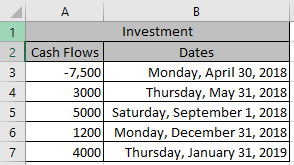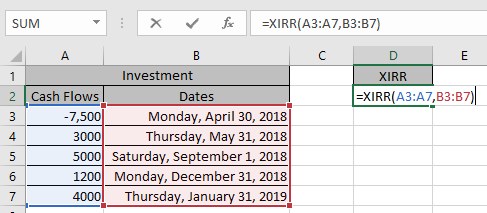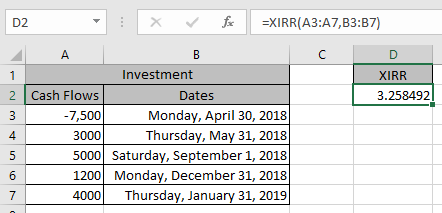# How to use the Excel XIRR function

In this article, we will learn how to get the Interest rate of return for irregular interval using the XIRR function in Excel.

XIRR function returns the rate of interest of the Cashflow at irrrgular intervals unlike IRR function . It takes the argument as an array of Cash flow at an irregular interval given with dates.
Syntax:

=XIRR(Values, dates, [guess])

Values: Array of Cash flow at regular intervals
Dates: Dates corresponding to cash flows
[guess]: [optional] Expected IRR. Default is .1 (10%)

Let’s learn this through an example shown below.
Here we have Cash flow record with dates along with it which are at irregualr intervals. And we need to find the rate of interest for the data.Use the formula

=XIRR( A3:A7 , B3:B7 )Press EnterThis 3.25% is the rate of return of the cashflow data.

Hope you learned how to use XIRR function in Excel. Explore more articles here on Accounting. Feel free to state your queries below. We will help you.

Related Articles:

How to Use IRR function in Excel

How to use the MIRR function in Excel

How to Use RATE Function in Excel

Popular Articles:

50 Excel Shortcuts to Increase Your Productivity

How to use the VLOOKUP Function in Excel

How to use the COUNTIF function in Excel 2016

How to Use SUMIF Function in Excel

Terms and Conditions of use

The applications/code on this site are distributed as is and without warranties or liability. In no event shall the owner of the copyrights, or the authors of the applications/code be liable for any loss of profit, any problems or any damage resulting from the use or evaluation of the applications/code.